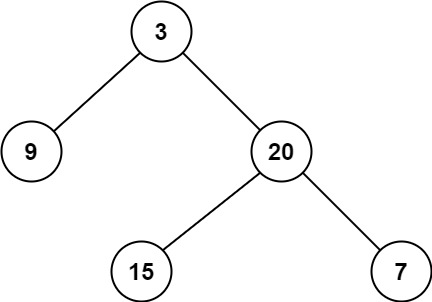Coding

# LeetCode Minimum Depth of Binary Tree Solution

0
(0)

Given a binary tree, find its minimum depth.

The minimum depth is the number of nodes along the shortest path from the root node down to the nearest leaf node.

Note: A leaf is a node with no children.

Example 1:```Input: root = [3,9,20,null,null,15,7]
Output: 2
```

Example 2:

```Input: root = [2,null,3,null,4,null,5,null,6]
Output: 5
```

Constraints:

• The number of nodes in the tree is in the range `[0, 105]`.
• `-1000 <= Node.val <= 1000`

Solution

We need to find minimum depth of binary tree. At first, I thought this problem would be very simple and I will simply solve it using recursion. But after a lot of trials and errors I could not find the right solution. It was always failing for use cases of skewed binary trees. While I was almost sure that the solution would be a DFS recursion approach, I could not simply derive it on my own.

After some time I looked at the solution. To solve this, we have to do the following. If the root is null/empty, then obviously depth is zero. Calculate minDepth of left and right subtree recursively. If either of them is zero, that means your node has only one child, so you have to calculate depth of that one child and add one to it. If both of them are non zero, then return minimum of depth of right and left subtrees and add one two it. It is very important for you to understand the use case of when the node has only one child, we calculate depth of that one child only and add one to it. Once you have understood this logic, coding will be easy.

Below is the Java code for this approach.

`````` public int minDepth(TreeNode root) {
if (root == null) return 0;
int leftDepth = minDepth(root.left);
int rightDepth = minDepth(root.right);
if (leftDepth == 0 || rightDepth == 0) {
return Math.max(leftDepth, rightDepth) + 1;
}
else {
return Math.min(leftDepth, rightDepth) + 1;
}
}``````

And below is the efficiency of this solution on LeetCode.Definitely as you can see above, there is a scope of improvement for this solution. BFS approach could be faster. Why don’t you go ahead and try a BFS approach to solve this problem.

Lessons Learnt

Some problems look simple and easy but they are not. This problem falls in that category. After giving a very hard try at the problem, then when you look at the solution you will understand it in an instant. 🙂 And you will remember it for life.

Click on a star to rate it!

Average rating 0 / 5. Vote count: 0

No votes so far! Be the first to rate this post.

As you found this post useful...

Follow us on social media!

We are sorry that this post was not useful for you!

Let us improve this post!

Tell us how we can improve this post?